GFG App
Open AppBrowser
Continue

# Checkbox in MATLAB App Building

MATLAB app builder allows you to create GUI apps without having proper software development knowledge. Matlab helps you create professional apps by just drag and dropping visuals according to your applications and writing code for its behavior. Later on, you can share your app either by sharing MATLAB drive or by creating standalone desktop and web apps with MATLAB Compiler. There are so many components available in Matlab App Builder.

In a user interface application, sometimes it is required to provide a list of options from which the user has to select any number of choices. This type of user interface(UI) component is called the Checkbox. It is basically a small square box where the user has to select and unselect to provide their preferences. These UI controls allow users to make selections. Creating Checkbox in MATLAB app designer, the steps are:

Step 1: Open MATLAB and select the APPS menu and click Design App under File group.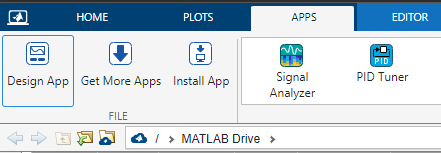Step 2:  Click the New option and select Blank app to create a new app. You can see so many components in the Component library placed on the left side and Component properties on the right-hand side.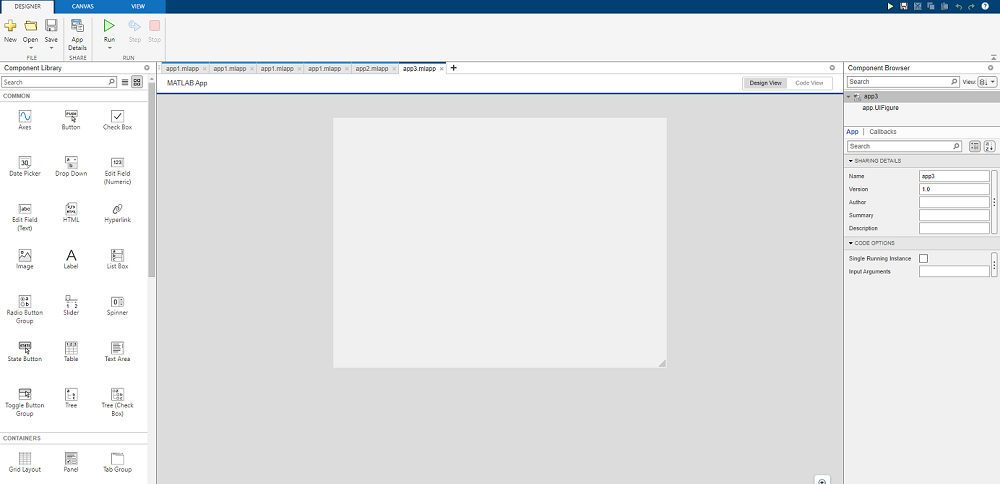Step 3:  Drag and drop Check Box from the Component Library on the figure provided on the canvas.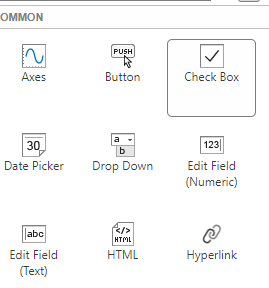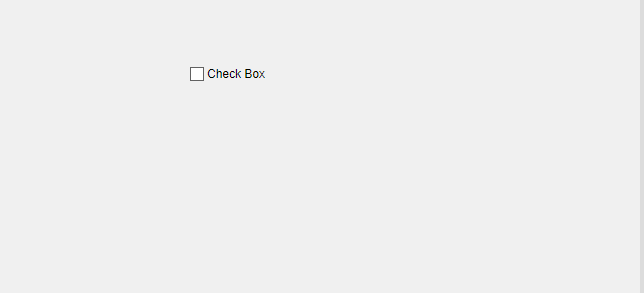Step 4:  A check box gets displayed on the figure. You can see different properties of the Check Box at the side bar.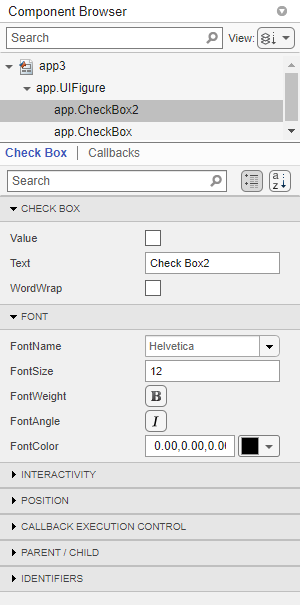The properties of Check Box are:

• CHECK BOX: It helps you to change the text , value (whether selected or unselected) of the check box.
• FONT: It helps you to change the font name, size, color, and angle of the check box text.
• INTERACTIVITY: It allows you to make the checkbox visible, enable or provide tooltip text that will display some text when you hover over to the checkbox.
• POSITION: It allows you to change the position of the checkbox on the figure.
• CALLBACK EXECUTION CONTROL: It controls incorruptibility and action.
• PARENT/ CHILD: It handles visibility.
• IDENTIFIER: They are used to add tags to check box.

Step 5:  We have dragged 5 checkboxes and changed their labels as C, JAVA, Python, C++, and MATLAB.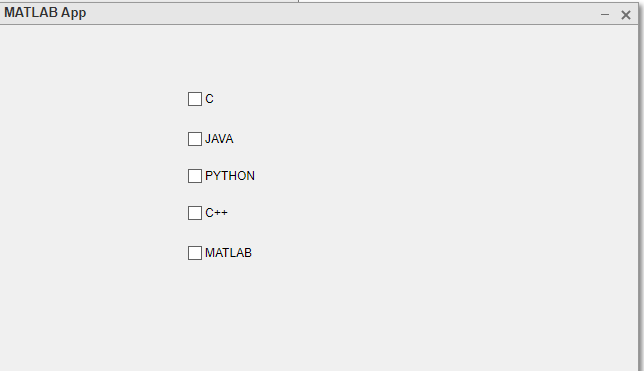After dragging and dropping the check box on the figure, we will write code to add functionality to it. So now, right-click on the first check box, i.e., C and select the Callbacks function, and click on Go to CCheckBoxValueChanged callback option.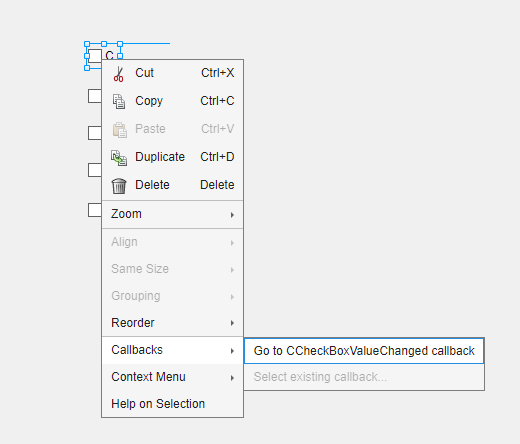Step 6:  It will take you to the code window, where it adds a function named CCheckBoxValueChanged ().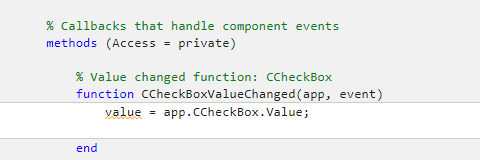Here, is the app. checkbox. The value attribute is used to give the status of the checkbox whether it is selected or not. The state of the checkbox is 0 for unselect and 1 for select. We can write the code in the space provided :

## Matlab

 `if` `value==1 ` `   ``fprintf(``"You have selected C language"``); ` `end`

we will write code for each checkbox following the steps given above.

Example:

## Matlab

 `  ``% MATLAB App code for GUI checkbox  ` `  ``classdef` `mycheckbox < matlab.apps.AppBase ` ` `  `    ``% Properties that correspond to app components ` `    ``properties (Access = public) ` `        ``UIFigure        matlab.ui.Figure ` `        ``MATLABCheckBox  matlab.ui.control.CheckBox ` `        ``CCheckBox_2     matlab.ui.control.CheckBox ` `        ``PYTHONCheckBox  matlab.ui.control.CheckBox ` `        ``JAVACheckBox    matlab.ui.control.CheckBox ` `        ``CheckBox2       matlab.ui.control.CheckBox ` `        ``CCheckBox       matlab.ui.control.CheckBox ` `    ``end` ` `  `    ``% Callbacks that handle component events ` `    ``methods (Access = private) ` ` `  `        ``% Value changed function: CCheckBox ` `        ``function` `CCheckBoxValueChanged(app, event) ` `            ``value = app.CCheckBox.Value; ` `            `  `            ``if` `value==1 ` `                ``fprintf(``"You have selected C language"``); ` `         `  `            ``end` ` `  `            `  `        ``end` ` `  `        ``% Value changed function: JAVACheckBox ` `        ``function` `JAVACheckBoxValueChanged(app, event) ` `            ``value = app.JAVACheckBox.Value; ` `            ``if` `value==1 ` `                ``fprintf(``"You have selected JAVA Language"``); ` `            `  `            ``end`    `        ``end` ` `  `        ``% Value changed function: PYTHONCheckBox ` `        ``function` `PYTHONCheckBoxValueChanged(app, event) ` `            ``value = app.PYTHONCheckBox.Value; ` `            ``if` `value==1 ` `                ``fprintf(``"You have selected Python language"``); ` `            ``end` `        ``end` ` `  `        ``% Value changed function: CCheckBox_2 ` `        ``function` `CCheckBox_2ValueChanged(app, event) ` `            ``value = app.CCheckBox_2.Value; ` `            ``if` `value==1 ` `                ``fprintf(``"You have selected C++ language"``); ` `            ``end` `        ``end` ` `  `        ``% Value changed function: MATLABCheckBox ` `        ``function` `MATLABCheckBoxValueChanged(app, event) ` `            ``value = app.MATLABCheckBox.Value; ` `            ``if` `value==1 ` `                ``fprintf(``"You have selected MATLAB"``); ` `            ``end` `        ``end` `    ``end` ` `  `    ``% Component initialization ` `    ``methods (Access = private) ` ` `  `        ``% Create UIFigure and components ` `        ``function` `createComponents(app) ` ` `  `            ``% Create UIFigure and hide until all components are created ` `            ``app.UIFigure = uifigure(``'Visible'``, ``'off'``); ` `            ``app.UIFigure.Position = [100 100 640 480]; ` `            ``app.UIFigure.Name = ``'MATLAB App'``; ` ` `  `            ``% Create CCheckBox ` `            ``app.CCheckBox = uicheckbox(app.UIFigure); ` `            ``app.CCheckBox.ValueChangedFcn = createCallbackFcn(app, ` `            ``@CCheckBoxValueChanged, true); ` `            ``app.CCheckBox.Text = ``'C'``; ` `            ``app.CCheckBox.Position = [191 396 31 22]; ` ` `  `            ``% Create CheckBox2 ` `            ``app.CheckBox2 = uicheckbox(app.UIFigure); ` `            ``app.CheckBox2.Text = ``'Check Box2'``; ` `            ``app.CheckBox2.Position = [958 74 87 22]; ` ` `  `            ``% Create JAVACheckBox ` `            ``app.JAVACheckBox = uicheckbox(app.UIFigure); ` `            ``app.JAVACheckBox.ValueChangedFcn = createCallbackFcn(app,  ` `            ``@JAVACheckBoxValueChanged, true); ` `            ``app.JAVACheckBox.Text = ``'JAVA'``; ` `            ``app.JAVACheckBox.Position = [191 356 50 22]; ` ` `  `            ``% Create PYTHONCheckBox ` `            ``app.PYTHONCheckBox = uicheckbox(app.UIFigure); ` `            ``app.PYTHONCheckBox.ValueChangedFcn = createCallbackFcn(app, ` `            ``@PYTHONCheckBoxValueChanged, true); ` `            ``app.PYTHONCheckBox.Text = ``'PYTHON'``; ` `            ``app.PYTHONCheckBox.Position = [191 319 72 22]; ` ` `  `            ``% Create CCheckBox_2 ` `            ``app.CCheckBox_2 = uicheckbox(app.UIFigure); ` `            ``app.CCheckBox_2.ValueChangedFcn = createCallbackFcn(app,  ` `            ``@CCheckBox_2ValueChanged, true); ` `            ``app.CCheckBox_2.Text = ``'C++'``; ` `            ``app.CCheckBox_2.Position = [191 282 45 22]; ` ` `  `            ``% Create MATLABCheckBox ` `            ``app.MATLABCheckBox = uicheckbox(app.UIFigure); ` `            ``app.MATLABCheckBox.ValueChangedFcn = createCallbackFcn(app, ` `            ``@MATLABCheckBoxValueChanged, true); ` `            ``app.MATLABCheckBox.Text = ``'MATLAB'``; ` `            ``app.MATLABCheckBox.Position = [191 242 69 22]; ` ` `  `            ``% Show the figure after all components are created ` `            ``app.UIFigure.Visible = ``'on'``; ` `        ``end` `    ``end` ` `  `    ``% App creation and deletion ` `    ``methods (Access = public) ` ` `  `        ``% Construct app ` `        ``function` `app = mycheckbox ` ` `  `            ``% Create UIFigure and components ` `            ``createComponents(app) ` ` `  `            ``% Register the app with App Designer ` `            ``registerApp(app, app.UIFigure) ` ` `  `            ``if` `nargout == 0 ` `                ``clear app ` `            ``end` `        ``end` ` `  `        ``% Code that executes before app deletion ` `        ``function` `delete(app) ` ` `  `            ``% Delete UIFigure when app is deleted ` `            ``delete(app.UIFigure) ` `        ``end` `    ``end` `end` `        `  `        `

Step 7: Click the Run button to run the code.

Output: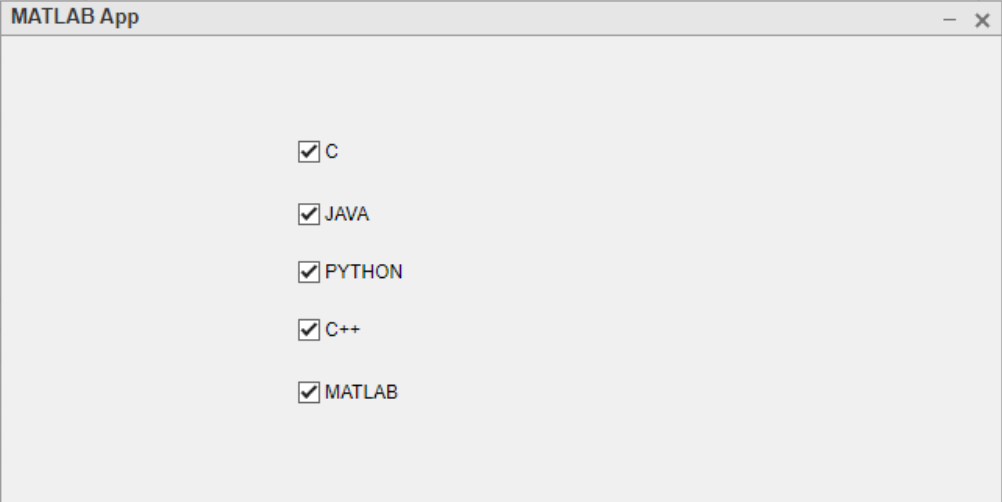My Personal Notes arrow_drop_up